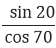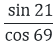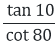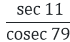# RD Sharma Class 10 Ex 5.3 Solutions Chapter 5 Trigonometric Ratios

In this chapter, we provide RD Sharma Class 10 Ex 5.3 Solutions Chapter 5 Trigonometric Ratios for English medium students, Which will very helpful for every student in their exams. Students can download the latest RD Sharma Class 10 Ex 5.3 Solutions Chapter 5 Trigonometric Ratios pdf, Now you will get step by step solution to each question.

# Chapter 5: Trigonometric Ratios Exercise – 5.3

### Question: 1

Evaluate the following:

### Solution:

(i) Given that,Since sin (90 – θ) = cos θ

Therefore,

(ii) Given that,

Since cos (90 – θ) = sin θ

Therefore

(iii) Given that,Since (90 – θ) = cos θ

(iv) We are given that,Since tan (90 – θ) = cot θ

Therefore

(v) Given that,Since sec (90 – θ) = cosec θ

Therefore

### Question: 2

Evaluate the following:

(ii) cos 48°- sin 42°

(vii) cosec 31° – sec 59°.

(viii) (sin 72° + cos 18°) (sin 72° – cos 18°)

(ix) sin 35° sin 55° – cos 35° cos 55°

(x) tan 48° tan 23° tan 42° tan 67°

(xi) sec 50° sin 40° + cos 40° cosec 50°

### Solution:

(i) We have to find:

sin(90° – θ) = cos θ and cos(90°- θ) = sin θ

= 1 + 1

= 2

(ii) We have to find: cos 48° – sin 42°

Since cos (90° − θ) = sin θ.

So cos 48° – sin 42° = cos (90° − 42°) – sin 42° = sin 42° – sin 42°= 0

So value of cos 48° – sin 42° is 0

(iii) We have to find:

Since cot (90°- θ) = tan θ and cos (90°- θ) = sin θ

(iv) We have to find:

Since sin(90°- θ) = cos θ and cos (90 – θ)

= 1 – 1

= 0

(v) We have to find:

Since tan (90°- θ) = cot θ and cot (90°- θ) = tan θ

= 1

(vi) We have to find:

= 1 + 1 = 2

(vii) We have to find:

cosec 31° − sec 59°

Since cosec (90° − θ) = sec θ.

So = cosec 31° − sec 59°

= cosec (90° − 59°) – sec 59° = sec 59° − sec 59° = 0

So value of cosec 31° − sec 59° is 0

(viii) We have to find:

(sin 72° + cos 18°)(sin 72° − cos 18°)

Since sin(90° − θ) = cos θ

So (sin 72° + cos 18°) (sin 72° − cos 18°)

= (sin 72°)2 – (cos 18°)2 = [sin(90° − 18°)]2 − (cos18°)2

= (cos18°)2 – (cos18°)2

= cos218°−cos218° = 0

So value of (sin 72° + cos 18°) (sin 72° − cos 18°) is 0.

(ix) We find:

sin 35°sin 55° – cos 35°cos 55°

Since sin(90° − θ) = cos θ and cos(90° − θ) = sin θ sin 35°sin 55°-  cos 35°cos 55°

= sin (90° − 55°) sin 55° – cos(90° − 55°) cos 55°

= 1 – 1

= 0

So value of sin 35° sin 55° – cos 35° cos 55° is 0

(x) We have to find:

tan 48° tan 23° tan 42° tan 67°

Since tan(90°− θ) = cot θ. So tan 48° tan 23° tan 42° tan 67°

= tan (90° − 42°) tan (90° − 67°) tan 42° tan67°

= cot 42°cot 67° tan 42° tan 67°

= (tan 67°cot 67°) (tan 42°cot 42°)

= 1 x 1 = 1

So value of tan 48° tan 23° tan 42° tan 67° is 1

(xi) We have to find:

sec 50° sin 40° + cos 40°cosec 50°

Since cos (90° − θ) = sin θ, sec (90°- θ) = cosec θ and sin θ. cosec θ = 1.

So sec 50° sin 40° + cos 40° cosec 50°

= sec (90° − 40°) sin 40° + cos (90° − 50°) cosec 50°

=1 + 1

= 2

So value of sec 50°sin 40° + cos 40°cosec 50° is 2.

### Question: 3

Express cos 75°+ cot 75° in terms of angle between 0° and 30°

### Solution:

Given that: cos 75° + cot 75°

= cos 75° + cot 75° = cos (90° − 15°) + cot (90° − 15°)

= sin 15° + tan 15°

Hence the correct answer is sin 15° + tan 15°

### Question: 4

If sin 3A = cos (A – 26°), where 3A is an acute angle, find the value of A.

### Solution:

We are given 3A is an acute angle. We have: sin 3A = cos (A – 26°)

⇒ sin 3A = sin(90° – (A – 26°))

⇒ sin3A = sin(116° – A)

⇒ 3A =116° – A

⇒ 4A = 116°

⇒ A = 29°

Hence the correct answer is 29°

### Question: 5

If A, B, C are the interior angles of a triangle ABC, prove that,

### Solution:

(i)  We have to prove:

Since we know that in triangle ABC

A + B + C = 180

⇒ C + A = 180° – B

Hence proved

(ii) We have to prove:

Since we know that in triangle ABC

A + B + C = 180

⇒ B + C = 180° – A

Hence proved

### Question: 6

Prove that:

(i) tan 20° tan 35° tan 45° tan 55° tan 70° = 1

(ii) sin 48°. Sec 48° + cos 48°.cosec 42° = 2

### Solution:

(i) Therefore tan 20° tan 35° tan 45° tan 55° tan 70°

= tan (90° − 70°) tan (90° − 55°) tan 45°tan 55° tan70°

= cot 70°cot 55° tan 45° tan 55° tan 70°

= (tan 70°cot 70°)(tan 55°cot 55°) tan 45°

= 1 × 1 × 1 = 1

Hence proved

(ii) We will simplify the left hand side

Sin 48°. Sec 48° + cos 48°. Cosec 42°

= sin 48°. sec (90° − 48°) cos 48°. cosec (90° − 48°)

= sin 48°.cos 48° + cos 48°.sin 48°

= 1 + 1 = 2

Hence proved

(iii) We have,

So we will calculate left hand side

= 1 + 1 – 2

= 2 – 2

= 0

Hence proved

(iv) We have

We will simplify the left hand side

= 1 + 1

= 2

Hence proved

### Question: 7

If A, B, C are the interior of triangle ABC, show that

### Solution:

(i) A + B + C = 180°

B + C = 180° – A/2

LHS = RHS

### Question: 8

If 2θ + 45° and 30 − θ are acute angles, find the degree measure of θ satisfying sin (20 + 45°) = cos (30° + θ)

### Solution:

Here 2θ + 45° = sin (60° + θ)

We know that, ((90° − θ) = cos (θ)

= sin (2θ + 45°) = sin (90° − (30° – θ))

= sin (2θ + 45°) = sin (90° − 30° – θ)

= sin (2θ + 45°)

= sin (60° + θ)

On equating sin of angle of we get,

= 2θ + 45° = 60° + θ

= θ = 60° – 45°

= θ = 15°

### Question: 9

If θ is appositive acute angle such that sec θ = cosec 60°, find 2cos2θ − 1

### Solution:

We know that,

sec (90° − θ) = cosec2θ = sec θ

= sec (90° − 60°)

= θ = 30°

= 2cos2 θ − 1

= 2cos2 30 − 1

### Question: 10

If sin 3θ = cos (θ − 6°) where 3θ and θ – 6° acute angles, find the value of θ.

### Solution:

We have, sin 3θ = cos (θ – 6°) cos (90° + 3θ)

= cos (θ − 6°)

= 90° − 3θ = θ − 6°

− 3θ – θ = – 6° − 90°

− 4θ = – 96°

θ = – 96°/- 4

θ = 24°

### Question: 11

If sec 2A = cosec (A − 42°) where 2A is acute angle, find the value of A.

### Solution:

We know that sec (90 – 3θ) = cosec θ

sec 2A = sec (90 − (A − 42))

sec 2A = sec (90 − A + 42))

sec 2A = sec (132 − A)

Now equating both the angles we get

2A = 132 – A

3A = 132

A = 132/3

A = 44

All Chapter RD Sharma Solutions For Class10 Maths

I think you got complete solutions for this chapter. If You have any queries regarding this chapter, please comment on the below section our subject teacher will answer you. We tried our best to give complete solutions so you got good marks in your exam.